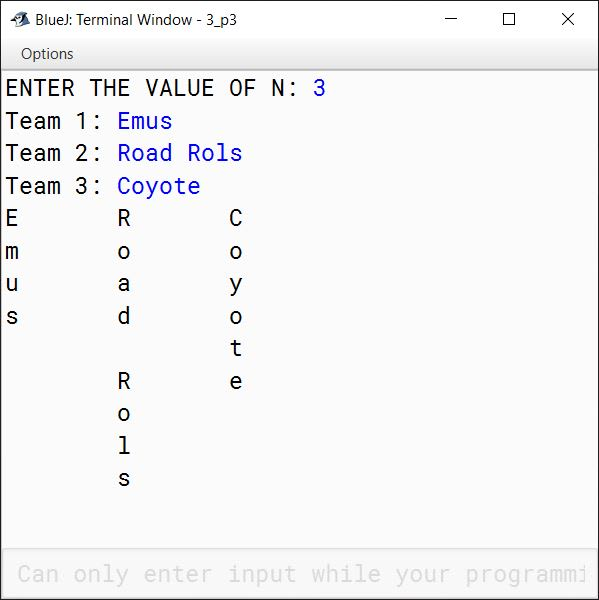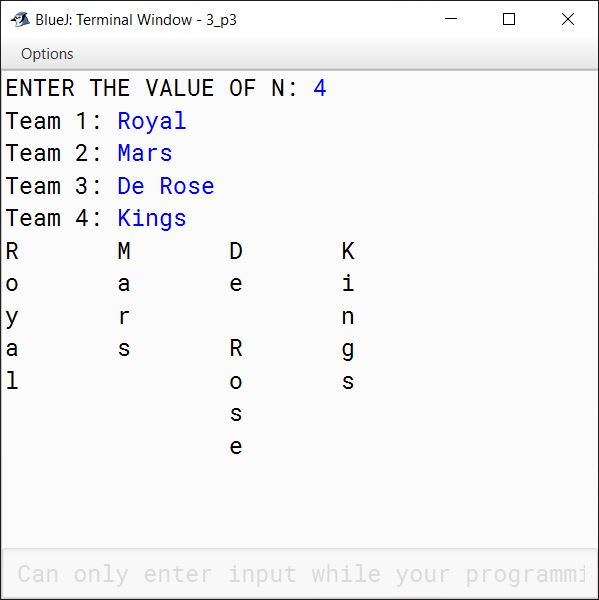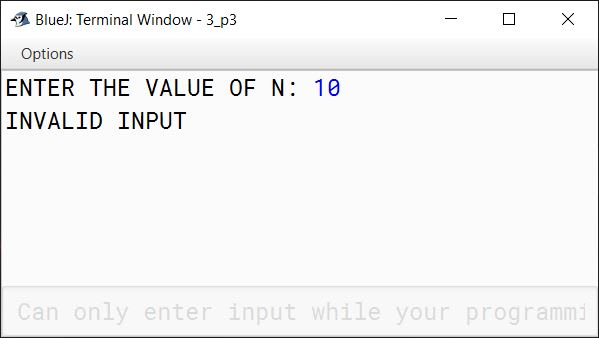# Solved 2018 Practical Paper ISC Computer Science

## Practical Questions

#### Question 1

A Goldbach number is a positive even integer that can be expressed as the sum of two odd primes.

Note: All even integer numbers greater than 4 are Goldbach numbers.

Example:

6 = 3 + 3
10 = 3 + 7
10 = 5 + 5

Hence, 6 has one odd prime pair 3 and 3. Similarly, 10 has two odd prime pairs, i.e. 3 and 7, 5 and 5.

Write a program to accept an even integer 'N' where N > 9 and N < 50. Find all the odd prime pairs whose sum is equal to the number 'N'.

Test your program with the following data and some random data:

Example 1

INPUT:
N = 14

OUTPUT:
PRIME PAIRS ARE:
3, 11
7, 7

Example 2

INPUT:
N = 30

OUTPUT:
PRIME PAIRS ARE:
7, 23
11, 19
13, 17

Example 3

INPUT:
N = 17

OUTPUT:
INVALID INPUT. NUMBER IS ODD.

Example 4

INPUT:
N = 126

OUTPUT:
INVALID INPUT. NUMBER OUT OF RANGE.

##### Solution
import java.util.Scanner;

public class GoldbachNumber
{
public static boolean isPrime(int num) {
int c = 0;

for (int i = 1; i <= num; i++) {
if (num % i == 0) {
c++;
}
}

return c == 2;
}

public static void main(String args[]) {
Scanner in = new Scanner(System.in);
System.out.print("ENTER THE VALUE OF N: ");
int n = in.nextInt();

if (n <= 9 || n >= 50) {
System.out.println("INVALID INPUT. NUMBER OUT OF RANGE.");
return;
}

if (n % 2 != 0) {
System.out.println("INVALID INPUT. NUMBER IS ODD.");
return;
}

System.out.println("PRIME PAIRS ARE:");

int a = 3;
int b = 0;
while (a <= n / 2) {
b = n - a;

if (isPrime(a) && isPrime(b)) {
System.out.println(a + ", " + b);
}

a += 2;
}
}
}
##### Output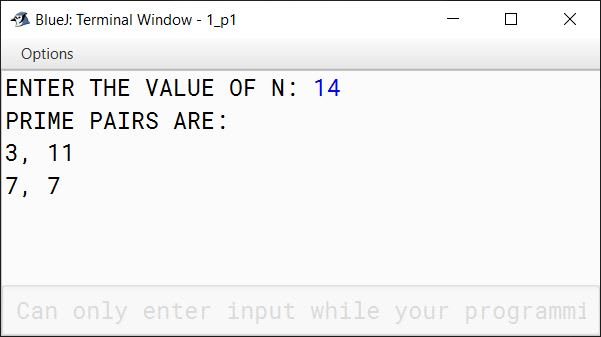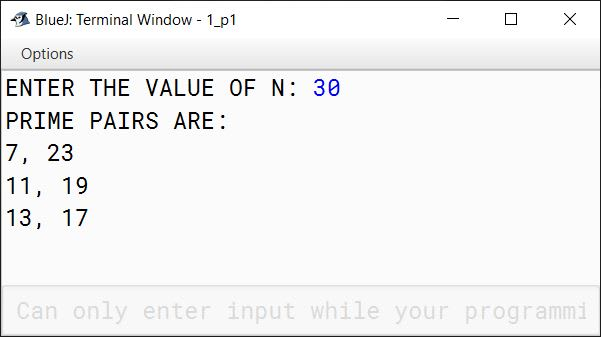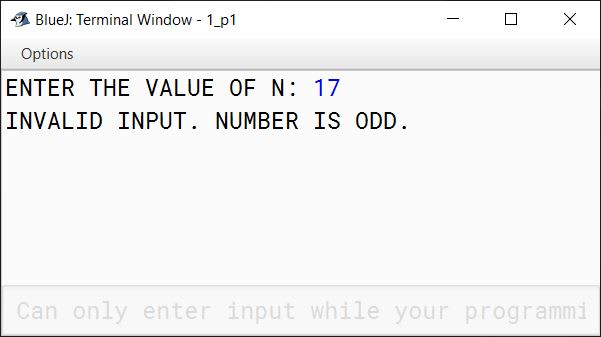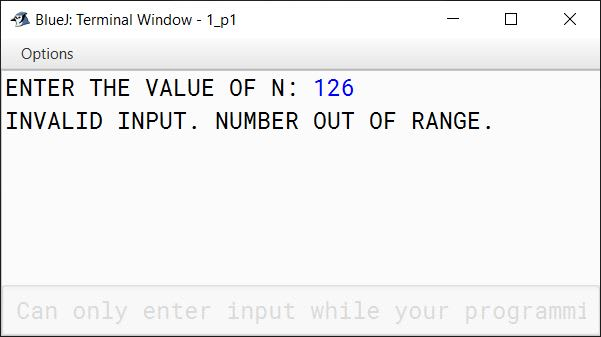#### Question 2

Write a program to declare a matrix a[][] of order (m × n) where 'm' is the number of rows and 'n' is the number of columns such that the values of both 'm' and 'n' must be greater than 2 and less than 10. Allow the user to input integers into this matrix. Perform the following tasks on the matrix:

1. Display the original matrix.
2. Sort each row of the matrix in ascending order using any standard sorting technique.
3. Display the changed matrix after sorting each row.

Test your program for the following data and some random data:

Example 1

INPUT:
M = 4
N = 3

ENTER ELEMENTS OF MATRIX:

$\begin{matrix} 11 & -2 & 3 \\ 5 & 16 & 7 \\ 9 & 0 & 4 \\ 3 & 1 & 8 \end{matrix}$

OUTPUT:
ORIGINAL MATRIX

$\begin{matrix} 11 & -2 & 3 \\ 5 & 16 & 7 \\ 9 & 0 & 4 \\ 3 & 1 & 8 \end{matrix}$

MATRIX AFTER SORTING ROWS

$\begin{matrix} -2 & 3 & 11 \\ 5 & 7 & 16 \\ 0 & 4 & 9 \\ 1 & 3 & 8 \end{matrix}$

Example 2

INPUT:
M = 3
N = 3

ENTER ELEMENTS OF MATRIX

$\begin{matrix} 22 & 5 & 19 \\ 7 & 36 & 12 \\ 9 & 13 & 6 \end{matrix}$

OUTPUT:
ORIGINAL MATRIX

$\begin{matrix} 22 & 5 & 19 \\ 7 & 36 & 12 \\ 9 & 13 & 6 \end{matrix}$

MATRIX AFTER SORTING ROWS

$\begin{matrix} 5 & 19 & 22 \\ 7 & 12 & 36 \\ 6 & 9 & 13 \end{matrix}$

Example 3

INPUT:
M = 11
N = 5

OUTPUT:
MATRIX SIZE OUT OF RANGE.

##### Solution
import java.util.Scanner;

public class ArraySort
{
public static void main(String args[]) {
Scanner in = new Scanner(System.in);
System.out.print("ENTER THE VALUE OF M: ");
int m = in.nextInt();
System.out.print("ENTER THE VALUE OF N: ");
int n = in.nextInt();

if (m <= 2
|| m >= 10
|| n <= 2
|| n >= 10) {
System.out.println("MATRIX SIZE OUT OF RANGE.");
return;
}

int a[][] = new int[m][n];
System.out.println("ENTER ELEMENTS OF MATRIX:");
for (int i = 0; i < m; i++) {
System.out.println("ENTER ELEMENTS OF ROW " + (i+1) + ":");
for (int j = 0; j < n; j++) {
a[i][j] = in.nextInt();
}
}

System.out.println("ORIGINAL MATRIX");
for (int i = 0; i < m; i++) {
for (int j = 0; j < n; j++) {
System.out.print(a[i][j] + " ");
}
System.out.println();
}

for (int i = 0; i < m; i++) {
for (int j = 0; j < n - 1; j++) {
for (int k = 0; k < n - j - 1; k++) {
if (a[i][k] > a[i][k + 1]) {
int t = a[i][k];
a[i][k] = a[i][k+1];
a[i][k+1] = t;
}
}
}
}

System.out.println("MATRIX AFTER SORTING ROWS");
for (int i = 0; i < m; i++) {
for (int j = 0; j < n; j++) {
System.out.print(a[i][j] + " ");
}
System.out.println();
}
}
}
##### Output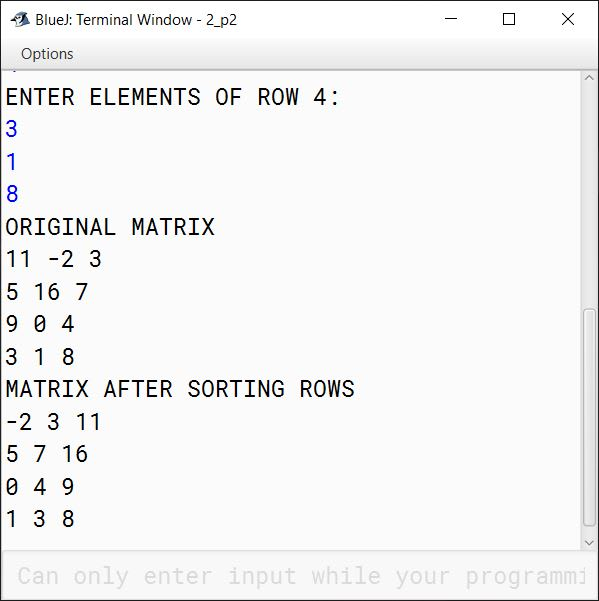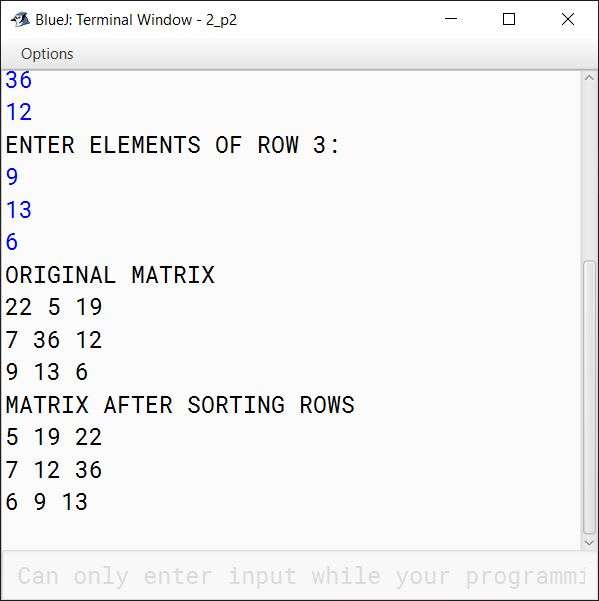#### Question 3

The names of the teams participating in a competition should be displayed on a banner vertically, to accommodate as many teams as possible in a single banner. Design a program to accept the names of N teams, where 2 < N < 9 and display them in vertical order, side by side with a horizontal tab (i.e. eight spaces).

Test your program for the following data and some random data:

Example 1

INPUT:
N = 3
Team 1: Emus
Team 3: Coyote

OUTPUT:
E        R       C
m       o       o
u        a        y
s        d        o
t
R        e
o
l
s

Example 2

INPUT:
N = 4
Team 1: Royal
Team 2: Mars
Team 3: De Rose
Team 4: Kings

OUTPUT:
R        M       D       K
o        a         e       i
y        r                  n
a        s          R      g
l                     o      s
s
e

Example 3

INPUT:
N = 10

OUTPUT:
INVALID INPUT

##### Solution
import java.util.Scanner;

public class Banner
{
public static void main(String args[]) {
Scanner in = new Scanner(System.in);
System.out.print("ENTER THE VALUE OF N: ");
int n = in.nextInt();
in.nextLine();

if (n <= 2 || n >= 9) {
System.out.println("INVALID INPUT");
return;
}

String teams[] = new String[n];
int highLen = 0;

for (int i = 0; i < n; i++) {
System.out.print("Team " + (i+1) + ": ");
teams[i] = in.nextLine();
if (teams[i].length() > highLen) {
highLen = teams[i].length();
}
}

for (int i = 0; i < highLen; i++) {
for (int j = 0; j < n; j++) {
int len = teams[j].length();
if (i >= len) {
System.out.print(" \t");
}
else {
System.out.print(teams[j].charAt(i) + "\t");
}
}
System.out.println();
}
}
}
##### Output